Question

5. A cereal factory’s machine is adjusted to fill boxes with a mean of 12 ounces and a standard deviation of 0.6 ounces. Assume the amount of fill is normally distributed.

a. In order to ensure boxes are not inappropriately filled, they need to be between the 45 and 55 percentiles. How many ounces should be in each box to ensure they fall within the appropriate fill level?

Given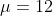and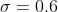Z-score of 45th percentile is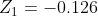and

Z-score of 55th percentile is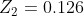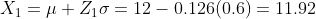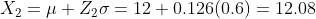#### Earn Coins

Coins can be redeemed for fabulous gifts.

Similar Homework Help Questions
• ### The mean weight of a box of cereal filled by a machine is 18.0 ounces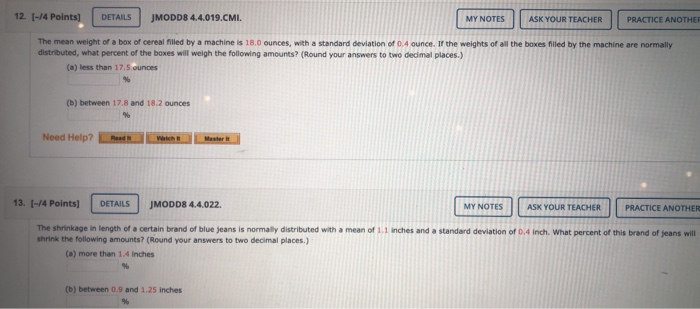The mean weight of a box of cereal filled by a machine is 18.0 ounces, with a standard deviation of 0.4 ounce. If the weights of all the boxes filled by the machine are normally distributed, what percent of the boxes will weigh the following amounts? (Round your answers to two decimal places.) (a) less than 17.5 ounces (b) between 17.8 and 18.2 ounces

• ### A machine fills 24-ounce (according to the label) boxes with cereal. The amount deposited into the...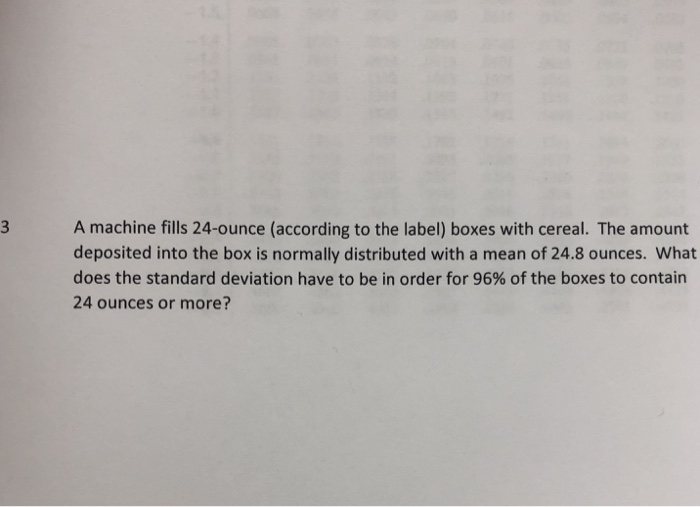A machine fills 24-ounce (according to the label) boxes with cereal. The amount deposited into the box is normally distributed with a mean of 24.8 ounces. What does the standard deviation have to be in order for 96% of the boxes to contain 24 ounces or more?

• ### A cereal box filling machine is designed to release an amount of 12 ounces of cereal...

A cereal box filling machine is designed to release an amount of 12 ounces of cereal into each box, and the machine’s manufacturer wants to know of any departure from this setting. The engineers at the factory randomly sample 100 boxes of cereal and find a sample mean of 12.25 ounces. If we know from previous research that the population is normally distributed with a standard deviation of 1.51 ounces, is there evidence that the mean amount of cereal in...

• ### 1. A cereal box filling machine is designed to release an amount of 12 ounces of...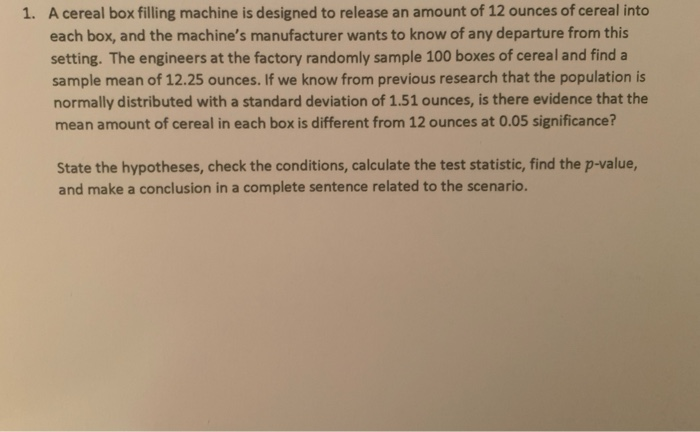1. A cereal box filling machine is designed to release an amount of 12 ounces of cereal into each box, and the machine's manufacturer wants to know of any departure from this setting. The engineers at the factory randomly sample 100 boxes of cereal and find a sample mean of 12.25 ounces. If we know from previous research that the population is normally distributed with a standard deviation of 1.51 ounces, is there evidence that the mean amount of cereal...

• ### The amount of cereal that can be poured into a small bowl is normally distributed with a mean of 1.5 ounces and a sta...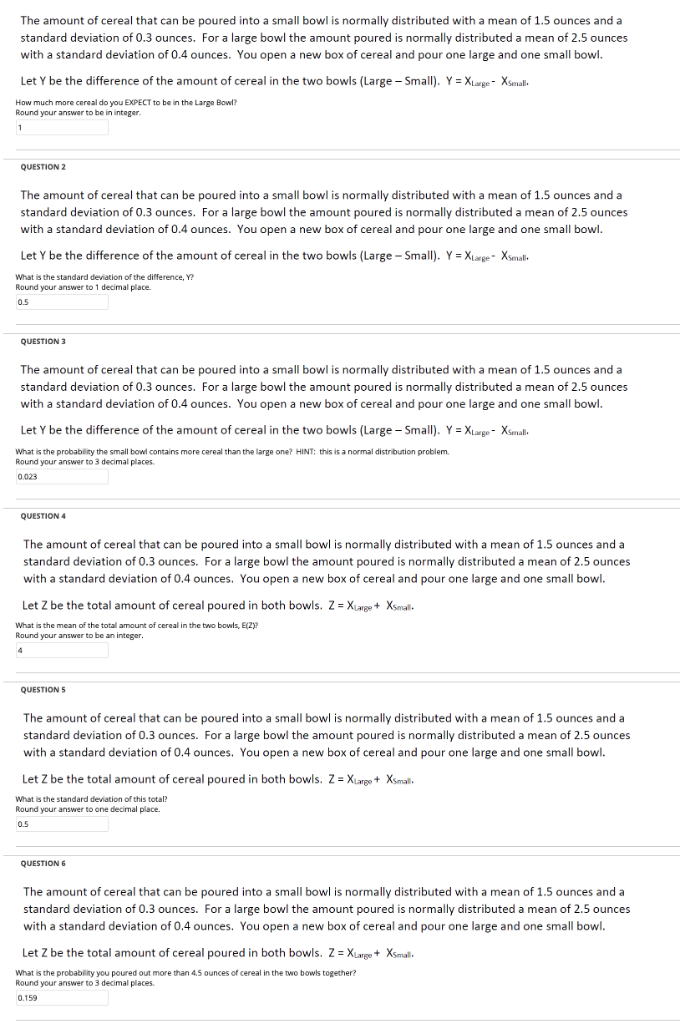The amount of cereal that can be poured into a small bowl is normally distributed with a mean of 1.5 ounces and a standard deviation of 0.3 ounces. For a large bowl the amount poured is normally distributed a mean of 2.5 ounces with a standard deviation of 0.4 ounces. You open a new box of cereal and pour one large and one small bowl. Let Y be the difference of the amount of cereal in the two bowls (Large-S...

• ### 58. Cereal A company's cereal boxes advertise that each box contains 9.65 ounces of cereal. In...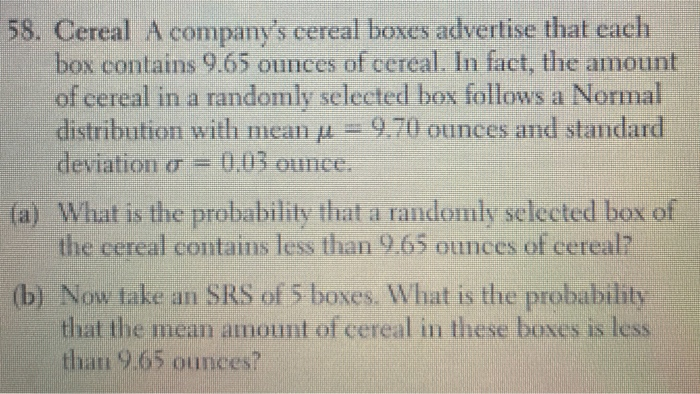58. Cereal A company's cereal boxes advertise that each box contains 9.65 ounces of cereal. In fact, the amount of cereal in a randomly selected box follows a Normal distribution with mean μ 9.70 ounces and standard deviation σ = 0,03 ounce. (a) What is the probability that a randomly selected box of the cereal contains less than 9.65 ounces of cereal? (b) Now take an SRS of 5 boxes. What is the probability that the mean amount of cereal...

• ### 2. A store sells "16-ounce" boxes of Captain Crisp cereal. A random sample of 12 boxes...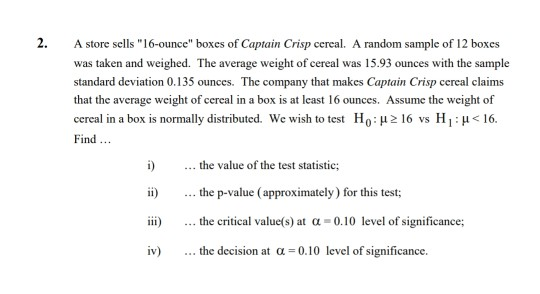2. A store sells "16-ounce" boxes of Captain Crisp cereal. A random sample of 12 boxes was taken and weighed. The average weight of cercal was 15.93 ounces with the sample standard deviation 0.135 ounces. The company that makes Captain Crisp cereal claims that the average weight of cereal in a box is at least 16 ounces. Assume the weight of cereal in a box is normally distributed. We wish to test Ho: μ 16 vs H 1 : μ...

• ### A store sells "16-ounce" boxes of Captain Crisp cereal. A random sample of 12 boxes was...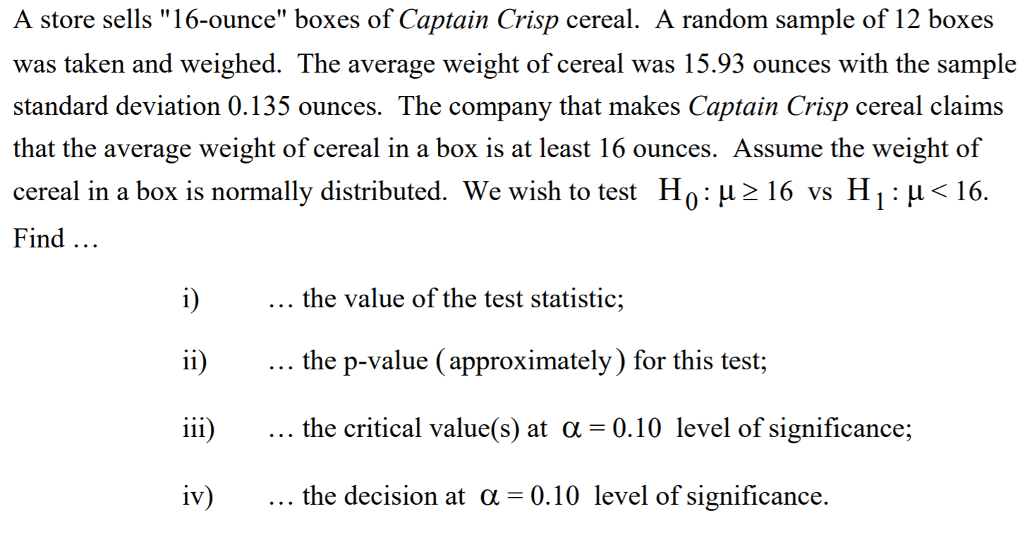A store sells "16-ounce" boxes of Captain Crisp cereal. A random sample of 12 boxes was taken and weighed. The average weight of cereal was 15.93 ounces with the sample standard deviation 0.135 ounces. The company that makes Captain Crisp cereal claims that the average weight of cereal in a box is at least 16 ounces. Assume the weight of cereal in a box is normally distributed. We wish to test H 0 : μ 16 vs H 1 :...

• ### The weights of boxes of Brand Z cereal were found to be normally distrubted with a mean of 16.5 ounces and a standard deviation of 0.4 ounces

The weights of boxes of Brand Z cereal were found to be normally distrubted with a mean of 16.5 ounces and a standard deviation of 0.4 ounces. a. what percentage of the boxes will weigh more than 16 ounces? b. what percentage of the boxes will weigh between 15.5 and 16.5 ounces? c. if a store has 500 boxes of cereal, how many of them will weigh less than 15.5 ounces

• ### The weights of boxes of Brand Z cereal were found to be normally distrubted with a mean of 16.5 ounces and a standard deviation of 0.4 ounces

The weights of boxes of Brand Z cereal were found to be normally distrubted with a mean of 16.5 ounces and a standard deviation of 0.4 ounces. a. what percentage of the boxes will weigh more than 16 ounces? b. what percentage of the boxes will weigh between 15.5 and 16.5 ounces? c. if a store has 500 boxes of cereal, how many of them will weigh less than 15.5 ounces?